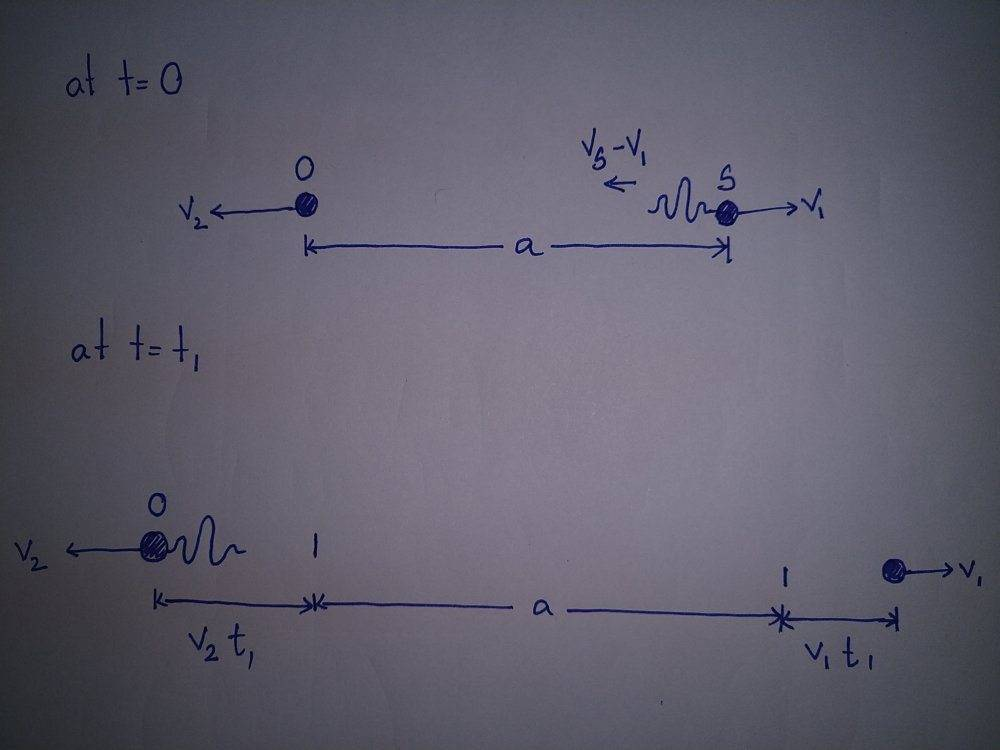# Time taken for sound to travel between two moving observers

Saptarshi Sarkar
Homework Statement:
A source S of frequency ##f_0## and an observer O, moving with speeds ##v_1## and ##v_2## respectively, are moving away from each other. When they are separated by distance a (t=0), a sound pulse is emitted by the source. Suppose velocity of sound to be ##v_s## and calculate the time ##t_1## that it takes for the pulse to be received by O.
Relevant Equations:
Total distance the pulse needs to travel:
##D = a + v_1t_1##

Speed of sound pulse = ##v_s - v_2##

So,
##t_1 = \frac {a + v_1t_1} {v_s - v_2}##
But the solution should be

##t_1 = \frac a {v_s - v_2}##

I assumed the following -

1. I did not consider the frequency as the Doppler shift in frequency was not asked.

2. I did not add the distance the source moved in time ##t_1## to the total distance traveled by the wave as the pulse was emitted at t=0.

Is any of my assumptions wrong?

Homework Helper
Gold Member
2022 Award
You could sketch the motions of source, observer and pulse on a distance-time graph.

•Saptarshi Sarkar
Homework Helper
Gold Member
2022 Award
So,
##t_1 = \frac {a + v_1t_1} {v_s - v_2}##

I assumed the following -

2. I did not add the distance the source moved in time ##t_1## to the total distance traveled by the wave as the pulse was emitted at t=0.

How is that equation based on your assumption?

Are taking ##v_2## to be the speed of the source?

•Saptarshi Sarkar
Saptarshi Sarkar
Sorry, I guess I messed up the velocities. I will edit the question and add a sketch tomorrow morning.

Saptarshi Sarkar
Can't edit the question, so posting it here

Homework Statement::

A source S of frequency ##f_0## and an observer O, moving with speeds ##v_1## and ##v_2## respectively, are moving away from each other. When they are separated by distance a (t=0), a sound pulse is emitted by the source. Suppose velocity of sound to be ##v_s## and calculate the time ##t_1## that it takes for the pulse to be received by O.
Homework Equations::

Total distance the pulse needs to travel:
##D = a + v_2t_1##

Speed of sound pulse = ##v_s - v_1##

So,
##t_1 = \frac {a + v_2t_1} {v_s - v_1}##

But the solution should be

##t_1 = \frac a {v_s - v_2}##

I assumed the following -

1. I did not consider the frequency as the Doppler shift in frequency was not asked.

2. I did not add the distance the source moved in time ##t_1## to the total distance traveled by the wave as the pulse was emitted at t=0.

Is any of my assumptions wrong?•